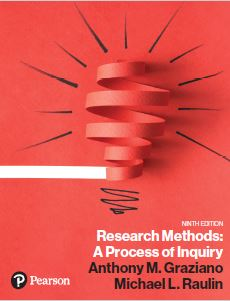﻿ Chapter 5 True/FalseGraziano & Raulin
Research Methods (9th edition)

## Chapter 5 True/False Questions Statistical Analysis of Data

Challenge yourself with these true/false questions. Click on your choice to see if you are correct.

1. The purpose of inferential statistics is to simplify and organize the data from a study. (True/False)

2. Individual differences can be expected for psychological variables such as intelligence, anxiety, and athletic ability. (True/False)

3. Frequency distributions are a subset of inferential statistics. (True/False)

4. Summary statistics are a subset of descriptive statistics. (True/False)

5. Mean scores are helpful in the interpretation of nominal data. (True/False)

6. Cross-tabulation is a useful way to describe the relationship between two nominal variables. (True/False)

7. It is possible to convert a grouped frequency distribution to a frequency distribution without referring back to the original data. (True/False)

8. It is possible to convert a frequency distribution to a grouped frequency distribution without referring back to the original data. (True/False)

9. When you are using a continuous variable, it is best to use a frequency distribution instead of a grouped frequency distribution. (True/False)

10. A frequency polygon can be used to compare distributions of scores from more than one group. (True/False)

11. Traditionally, the frequency of each score (or interval) is shown on the Y axis or ordinate of a frequency polygon. (True/False)

12. Skewed distributions usually show the familiar bell shape. (True/False)

13. The normal distribution is actually defined by a mathematical equation. (True/False)

14. A classroom test that is too difficult will produce a distribution that is negatively skewed. (True/False)

15. The range is a frequently used measure of central tendency. (True/False)

16. Although it is possible to have more than one mode in a distribution, there will always be just one mean. (True/False)

17. The mean is the score at the 50th percentile. (True/False)

18. The most frequently used measure of central tendency is the median, which is the arithmetic average of the scores. (True/False)

19. The variance, unlike the range, uses all the scores in its computation. (True/False)

20. If all of the scores in a distribution are increased by exactly five points, the range will increase by five points. (True/False)

21. A product-moment correlation of -1.00 means that there is no linear relationship between the variables. (True/False)

22. With ordinal data, the appropriate correlation to use is the Spearman rank-order correlation. (True/False)

23. Cross-tabulation for nominal data is the conceptual equivalent of scatter plots for score data. (True/False)

24. A nonlinear relationship is best indexed with a product-moment correlation. (True/False)

25. A circular scatter plot usually indicates a moderately strong positive relationship between the variables. (True/False)

26. A sample is a subset of the people or objects in a population. (True/False)

27. The null hypothesis states that there are no individual differences within the groups. (True/False)

28. If we are using a t-test and we reject the null hypothesis, we can be sure that the population means are different. (True/False)

29. Alpha is the level of Type II error that we can expect. (True/False)

30. If nothing else changes, decreasing alpha from .05 to .01 will increase the probability of a Type II error. (True/False)

31. If we have two groups and want to compare the means of the groups, we can use either a t-test or an analysis of variance. (True/False)

32. The sensitivity of a statistical procedure to the differences being sought is called the reliability of the procedure. (True/False)